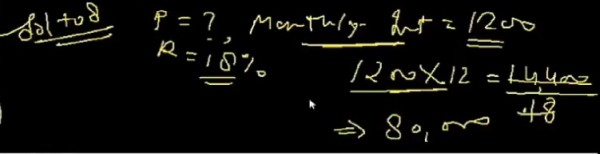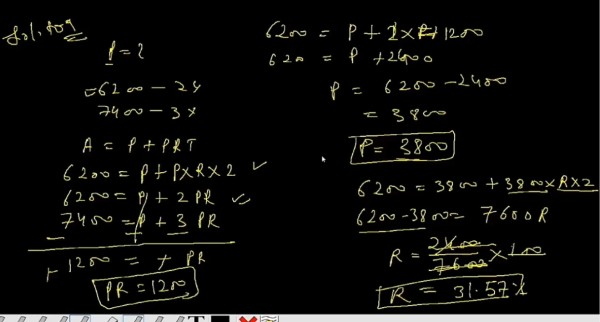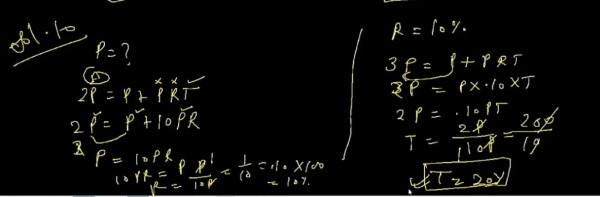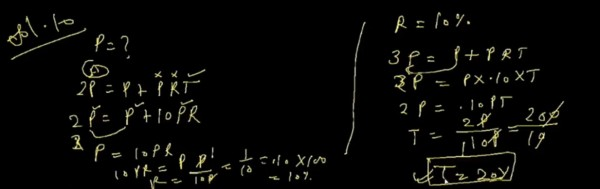CCI Online Learning
What do you want to learn today?

# Quantative aptitude problems

RishebhKumar (Studying)     16 May 2012

##### RishebhKumar
Studying
1 likes  128 points

| My Other Post

Simple Interest

Q1. The sum of required to earn a monthly interest of Rs.1200 at 18% per annum SI is……….?

Q2. A sum  of money amount to Rs.6200 in 2 years and Rs.7400 in 3 years.The principal and rate of interest are………..?

Q3. A sum of money double itself in 10 years. The number of years it would triple itself is………..?

Explain with solution plz....intermediate(ipc)course (no)     16 May 2012

##### intermediate(ipc)course
no
39 likes  1460 points

View Profile | My Other Post

1. si = p*n*i

=1200*12*0.18

=2592intermediate(ipc)course (no)     16 May 2012

##### intermediate(ipc)course
no
39 likes  1460 points

View Profile | My Other Post

2. 6200 = p*2/12*i----------------------------1

7400 = p*3/12*i

therefore,

6200+7400 = p*2/12*i+p*3/12*i

13600 = 1/6 pi +1/4 pi

=10/24 pi

pi = 13600*24/10

=32640(principal 32000 + interest 640)intermediate(ipc)course (no)     16 May 2012

##### intermediate(ipc)course
no
39 likes  1460 points

View Profile | My Other Post

3. 2*p*10/12*i = 3*p*n*i

or,5/3 *p*i = 3*p*i*n

or,9*n = 5

or,n=5/9=0.56=0.6.Tejaswi Kasturi (student-cpt)     16 May 2012

##### Tejaswi Kasturi
student-cpt
63 likes  417 points

View Profile | My Other Post

1. The interest rate is 18% per annum as such per month it is 18/12% i.e. 1.5%. as the interest per month is 1.5% i = p*r*n/100;

1200 = p*1.5/100 implies p = 1200*100/1.5 =80,000

2.the question doesn't specify whether the interest is compound or simple

if simple interest

A = P+I = P + P*r*n/100

for 2 years

6200 = P + P*r*2/100

for 3 years

7400 = P + P*r*3/100

subtracting first from second we get P*r/100 = 1200

substituting it in the first equation we get 6200-2*(P*r/100)=P

i.e. P = 6200 - 2*(1200) = 6200 - 2400 = 3800

r = 1200*100/3800 = 31.5% (approx)

if compound interest

P(1+r)^2 = 6200; P(1+r)^3 = 7400 dividing second by first we get (1+r) = 7400/6200

implies r = 1200/6200 = 19.35%

P = 6200/(1.1935)^2 = 4352.22

3. Again the question doesn't specify whether the Interest is simple or compound.

Simple Interest:

P+P*r*10/100 = 2P implies r= 10% (cancel P on both sides)

to triple the amount  P+ P*10*n/100 = 3P implies n = 20 i.e. 20 years

Compound Interest

P(1+r)^10 = 2P implies r = 7.17% (take logarithms on both sides)

(1.0717)^n = 3 n = 15.85 yearsUsman (Accounting)     25 October 2017

##### Usman
Accounting
24 points

View Profile | My Other Post

Principal amount 3800 and rate is 31.57% u r wringUsman (Accounting)     25 October 2017

##### Usman
Accounting
24 points

View Profile | My Other Post

Sum amount is 80000DKG Dexterous Zone (Institute)     09 October 2018

##### DKG Dexterous Zone
Institute
32 points

View Profile | My Other Posthttps://www.youtube.com/watch?v=HArV5iuEWGsDKG Dexterous Zone (Institute)     01 November 2018

##### DKG Dexterous Zone
Institute
32 points

View Profile | My Other Postview more »